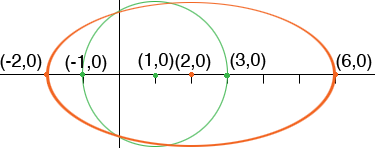SEARCH HOMEMath Central Quandaries & QueriesQuestion from bob, a student: I was wondering if you could double check my work? the question is as follows: The circle x^2+y^2-2x-3= 0 is stretched horizontally by a factor of 2 to obtain an ellipse what is the equation of this ellipse in general form? My work: (x^2-2x+1)+y^2=4 (x-1)^2+y^2=4 The ellipse will have a horizontal axis of 4 and vertical axis of 2. the semi-horizontal and semi-vertical axes are (2,1) therefore the equation is: (x-1)^2/2^2 + (y^2)/ (1^2) =1 is this correct?Hi Bob,

I agree with your rewriting of the equation x2+y2-2x-3 = 0 as (x-1)2+y2=4 since then it is clear that the equation represents the circle with centre (1,0) and radius 4. This is the green circle in my diagram.If you then stretched horizontally by a factor of 2 you multiply the x-values by 2. Thus the centre of the circle (1,0) moves to (2,0), the point (3,0) moves to (6,0) and the point (-1,0) moves to (-2,0) and I get the orange ellipse.

What is its equation?

PennyMath Central is supported by the University of Regina and The Pacific Institute for the Mathematical Sciences.